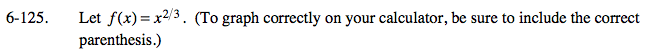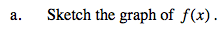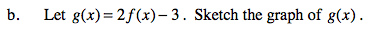### Home > PC > Chapter 6 > Lesson 6.3.2 > Problem6-125

6-125.
1. Let f(x) = x2/3. (To graph correctly on your calculator, be sure to include the correct parenthesis.) Homework Help ✎

1. Sketch the graph of f(x).

2. Let g(x) = 2f(x) − 3. Sketch the graph of g(x).